# Symmetry exercise 12.3 solutions class 7 NCERT

NCERT class 7 mathematics chapter 12 Symmetry Exercise 12.3 solutions are given.

You should practice all problems and solutions are given in the textbook.

Observe the given solutions and try them in your own method.

You can see.

NCERT maths class 7 solutions

Symmetry

Exercise 12.1

Exercise 12.2

Exercise 12.3

## Maths class 7 Symmetry exercise 12.3 solutions NCERT

Chapter 12

Symmetry

Exercise 12.3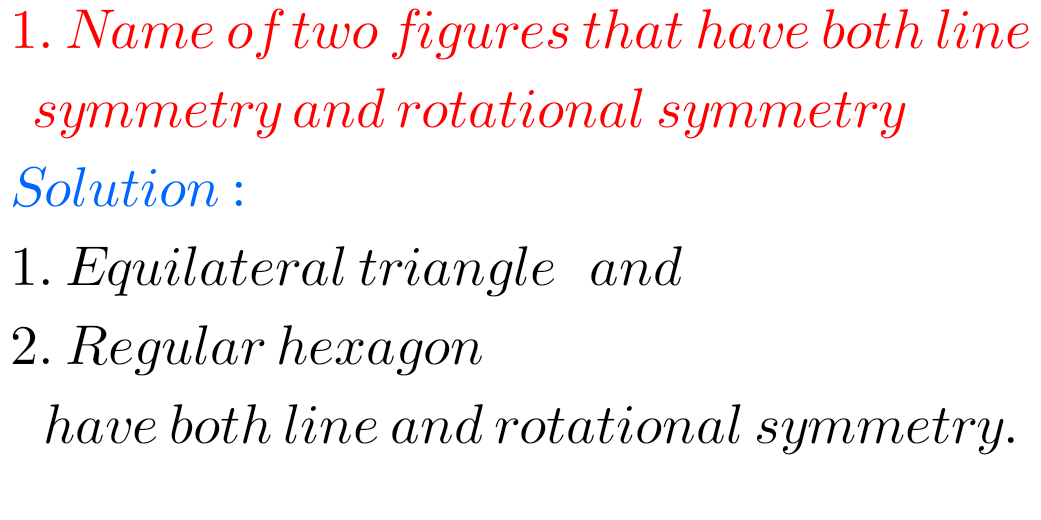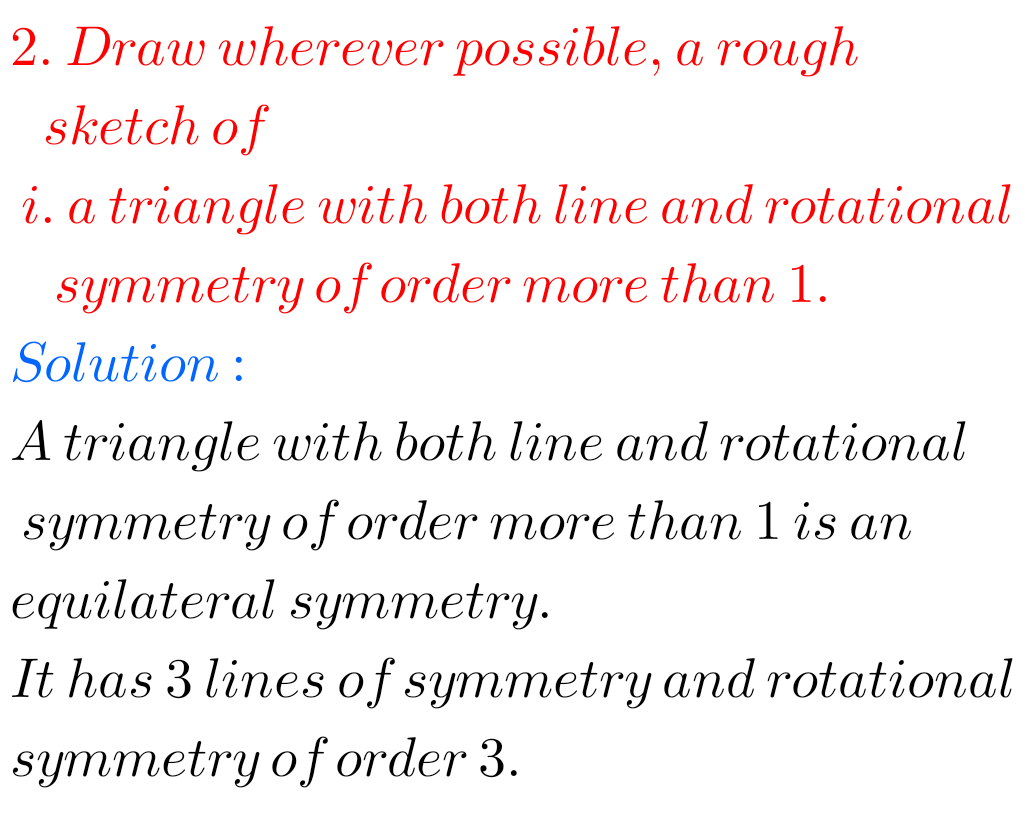FF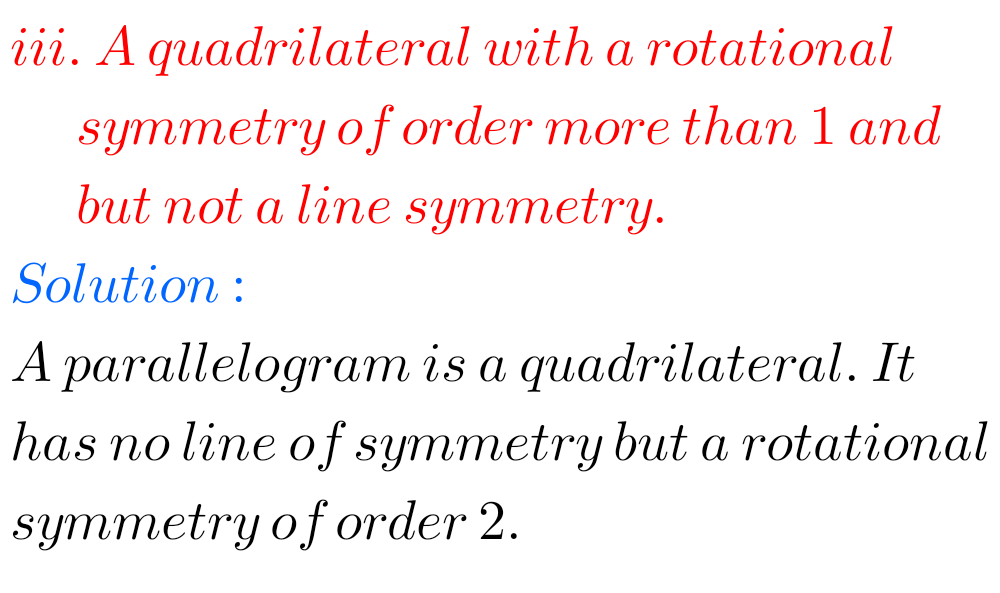F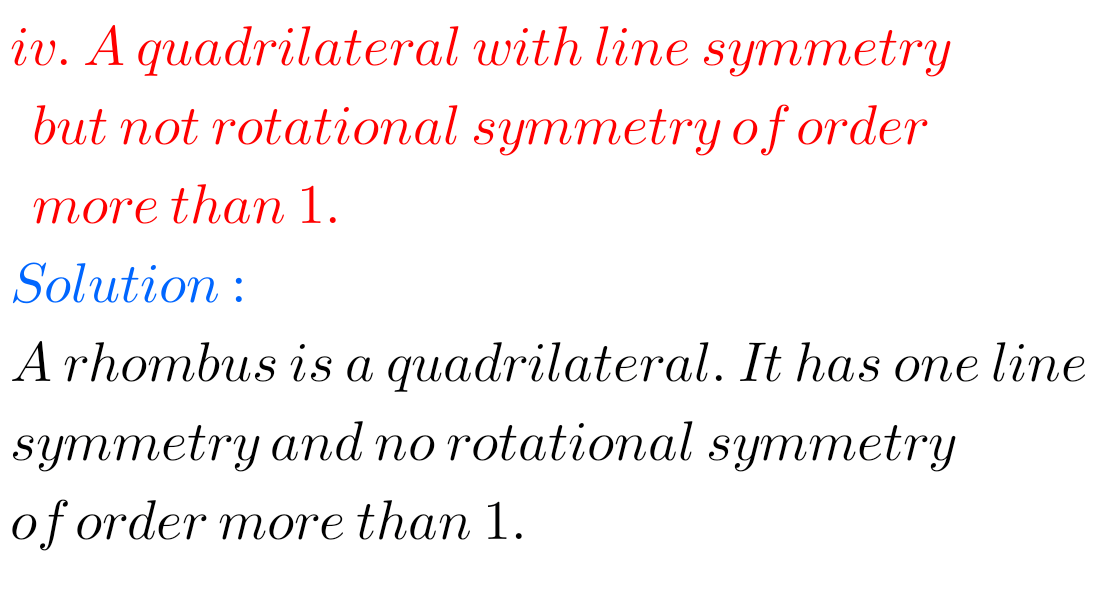F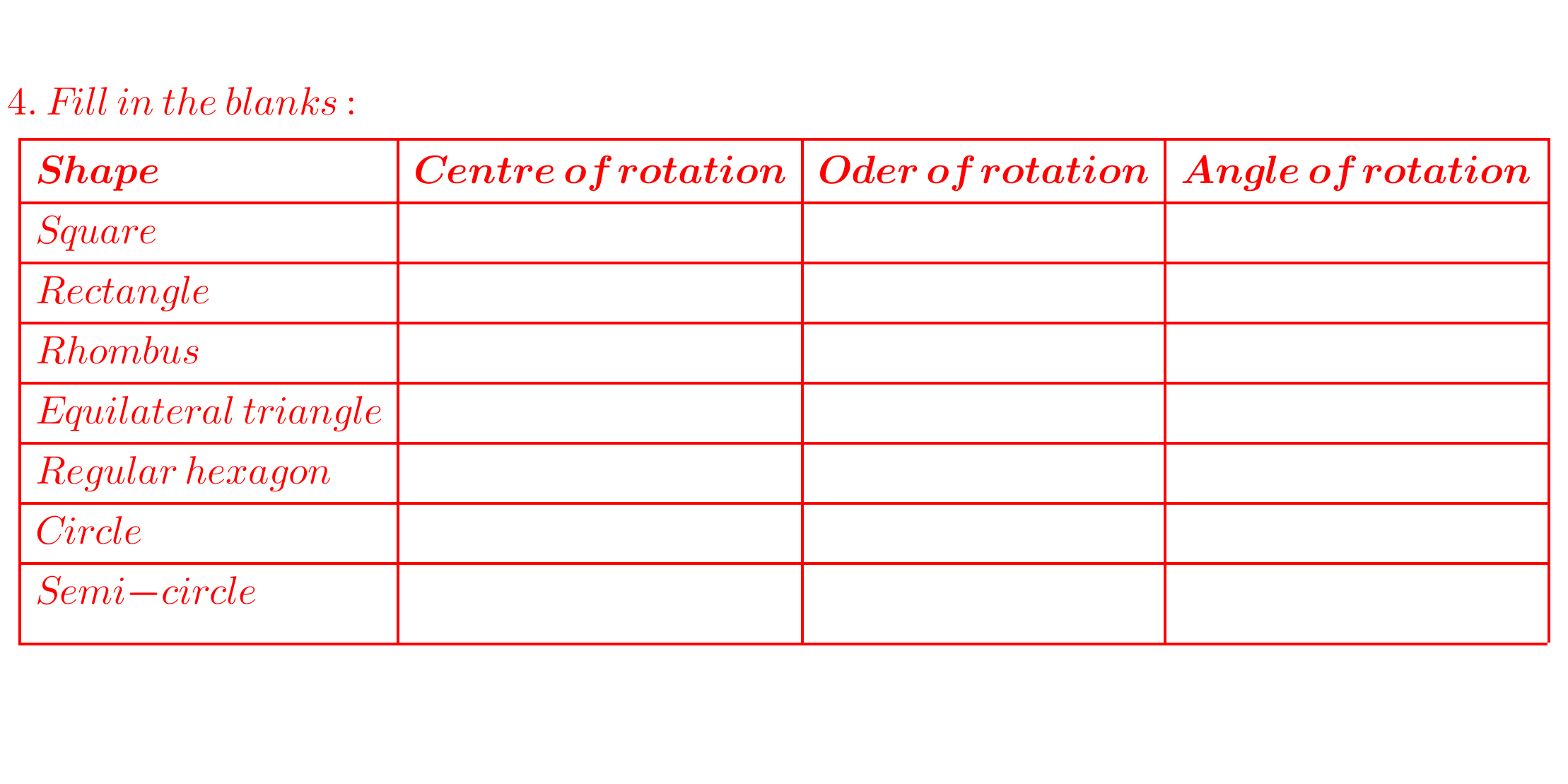### Solutions for exercise 12.3 Symmetry class 7 NCERT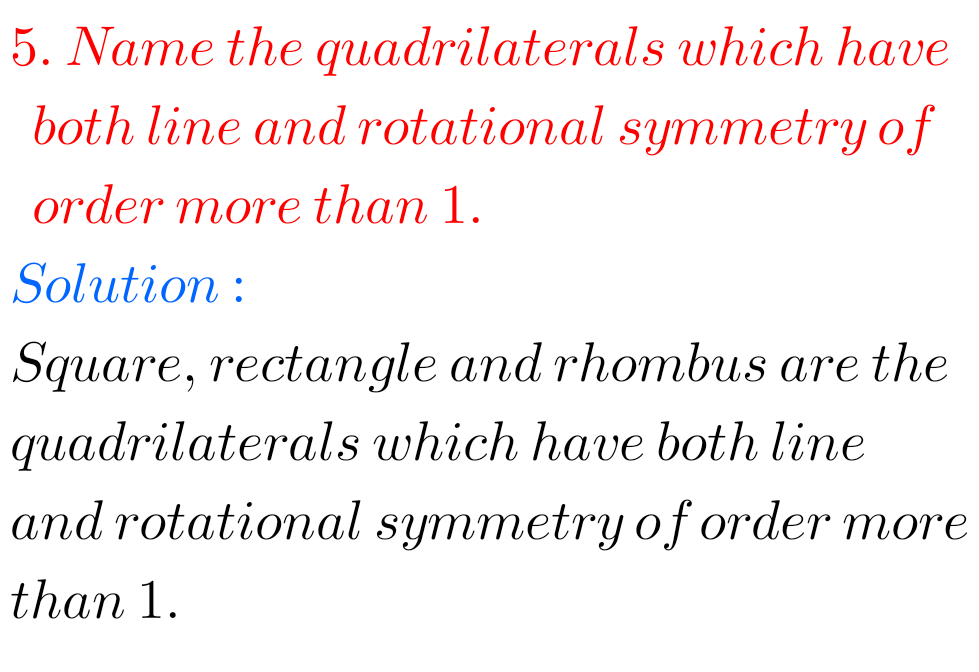FM

Note :Observe the solutions and try them in your own method.

NCERT maths class 7 solutions

NIOS Maths 311 book 2 solutions for some chapters

Inter Maths Solutions

SSC Maths solutions class 10

NCERT maths solutions class 6